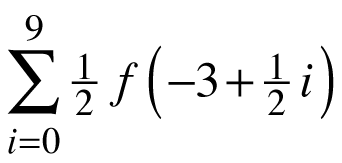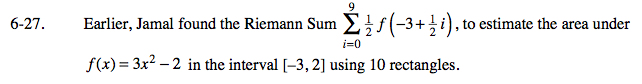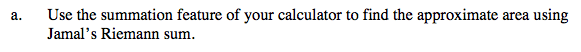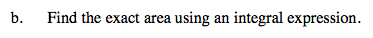### Home > CALC > Chapter 6 > Lesson 6.1.2 > Problem6-27

6-27.
1. Earlier, Jamal found the Riemann Sum, to estimate the area under f(x) = 3x2 − 2 in the interval [−3, 2] using 10 rectangles. Homework Help ✎

1. Use the summation feature of your calculator to find the approximate area using Jamal's Riemann sum.

2. Find the exact area using an integral expression.29.375 un²First set up an integral on the given interval.